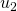# Arithmetic Sequences, Introduction

An arithmetic sequence is an ordered list – usually of numbers – where there is a common difference between terms.

Here is an example:Here is a second example:Notice that in these two examples, the common difference between terms is 3.

Number Sense Question 1:

If you continue these sequences indefinitely, will there ever be a common term?

Number Sense Question 2:

Create two arithmetic sequences such that there are common terms. Write out several terms of each sequence. Circle the terms that appear in both sequences. How far apart are they in each sequence? Why?

(For the next two applets, printing the pdf file Fill the gaps might be useful).

On the next applet, 3 of the first 7 terms of an arithmetic sequence are presented. Figure out the missing terms.

What method did you use to calculate the missing terms?

On these problems, two of the first seven terms of an arithmetic sequence are given. Figure out the first term.

What method did you use to find the first term?

Suppose we label the first term, the second term, and the seventh term. Can you write an expression forusingand? That is, a formula that will work every time you hit ‘new problem’ on this applet?